## Train Regression Trees Using Regression Learner App

This example shows how to create and compare various regression trees using the Regression Learner app, and export trained models to the workspace to make predictions for new data.

You can train regression trees to predict responses to given input data. To predict the response of a regression tree, follow the tree from the root (beginning) node down to a leaf node. At each node, decide which branch to follow using the rule associated to that node. Continue until you arrive at a leaf node. The predicted response is the value associated to that leaf node.

Statistics and Machine Learning Toolbox™ trees are binary. Each step in a prediction involves checking the value of one predictor variable. For example, here is a simple regression tree: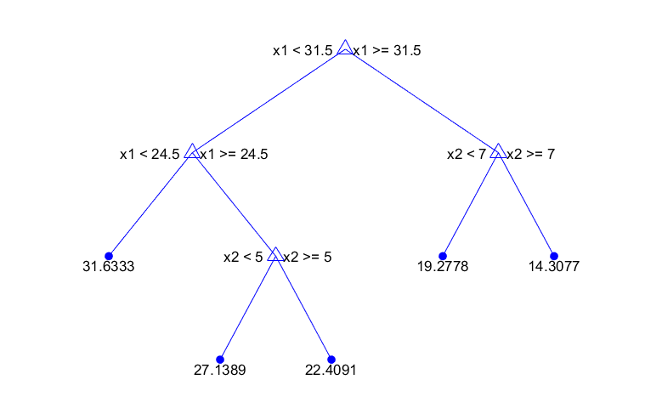This tree predicts the response based on two predictors, `x1` and `x2`. To predict, start at the top node. At each node, check the values of the predictors to decide which branch to follow. When the branches reach a leaf node, the response is set to the value corresponding to that node.

This example uses the `carbig` data set. This data set contains characteristics of different car models produced from 1970 through 1982, including:

• Acceleration

• Number of cylinders

• Engine displacement

• Engine power (Horsepower)

• Model year

• Weight

• Country of origin

• Miles per gallon (MPG)

Train regression trees to predict the fuel economy in miles per gallon of a car model, given the other variables as inputs.

1. In MATLAB®, load the `carbig` data set and create a table containing the different variables:

```load carbig cartable = table(Acceleration, Cylinders, Displacement,... Horsepower, Model_Year, Weight, Origin, MPG);```
2. On the Apps tab, in the Machine Learning and Deep Learning group, click Regression Learner.

3. On the Regression Learner tab, in the File section, select New Session > From Workspace.

4. Under Data Set Variable in the New Session from Workspace dialog box, select `cartable` from the list of tables and matrices in your workspace.

Observe that the app has preselected response and predictor variables. `MPG` is chosen as the response, and all the other variables as predictors. For this example, do not change the selections.5. To accept the default validation scheme and continue, click . The default validation option is cross-validation, to protect against overfitting.

Regression Learner creates a plot of the response with the record number on the x-axis.

6. Use the response plot to investigate which variables are useful for predicting the response. To visualize the relation between different predictors and the response, select different variables in the X list under X-axis.

Observe which variables are correlated most clearly with the response. `Displacement`, `Horsepower`, and `Weight` all have a clearly visible impact on the response and all show a negative association with the response.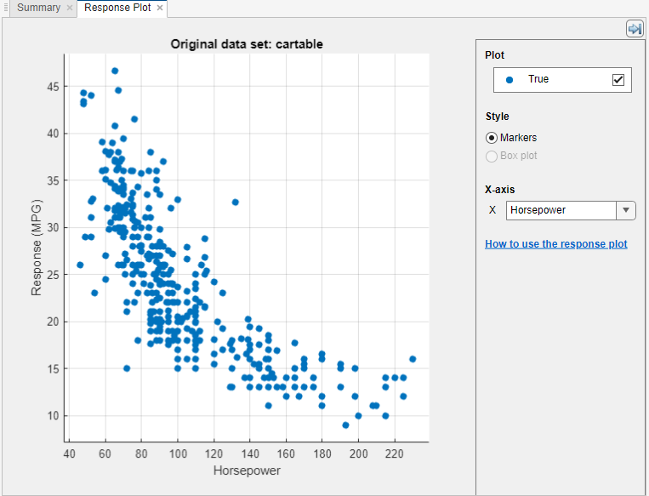7. Select the variable `Origin` under X-axis. A box plot is automatically displayed. A box plot shows the typical values of the response and any possible outliers. The box plot is useful when plotting markers results in many points overlapping. To show a box plot when the variable on the x-axis has few unique values, under Style, select Box plot.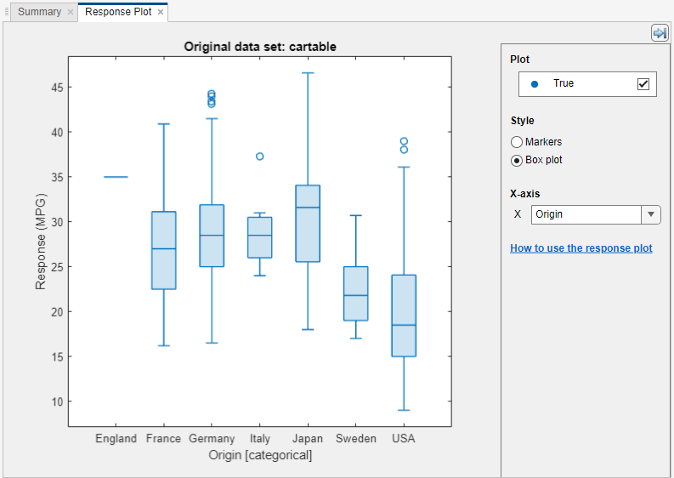8. Create a selection of regression trees. On the Regression Learner tab, in the Model Type section, click.

Then click.

Tip

If you have Parallel Computing Toolbox™, you can train all the models () simultaneously by selecting the button in the Training section before clicking . After you click , the Opening Parallel Pool dialog box opens and remains open while the app opens a parallel pool of workers. During this time, you cannot interact with the software. After the pool opens, the app trains the models simultaneously.

Regression Learner creates and trains three regression trees: a Fine Tree, a Medium Tree, and a Coarse Tree.

The three models appear in the Models pane. Check the RMSE (Validation) (validation root mean square error) of the models. The best score is highlighted in a box.

The Fine Tree and the Medium Tree have similar RMSEs, while the Coarse Tree is less accurate.

Regression Learner plots both the true training response and the predicted response of the currently selected model.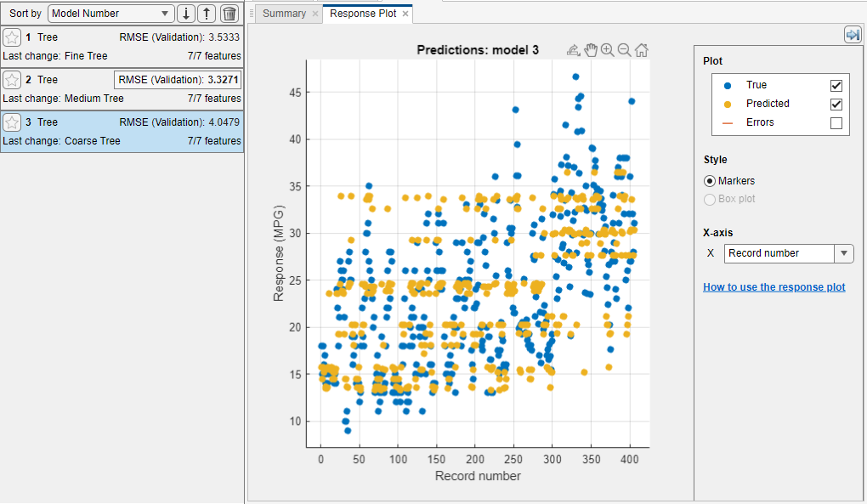Note

If you are using validation, there is some randomness in the results and so your model validation score can differ from the results shown.

9. Choose a model in the Models pane to view the results of that model. Under X-axis, select `Horsepower` and examine the response plot. Both the true and predicted responses are now plotted. Show the prediction errors, drawn as vertical lines between the predicted and true responses, by selecting the Errors check box.

10. See more details on the currently selected model in the Current Model Summary pane. Check and compare additional model characteristics, such as R-squared (coefficient of determination), MAE (mean absolute error), and prediction speed. To learn more, see View and Compare Model Statistics. In the Current Model Summary pane, you also can find details on the currently selected model type, such as options used for training the model.

11. Plot the predicted response versus true response. On the Regression Learner tab, in the Plots section, click Predicted vs. Actual and select Validation Data. Use this plot to understand how well the regression model makes predictions for different response values.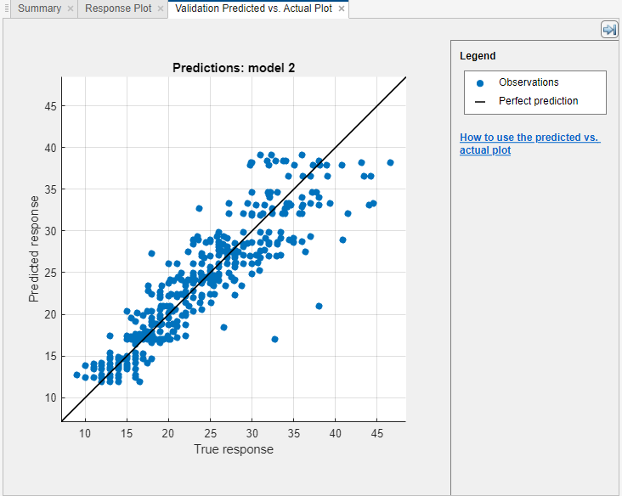A perfect regression model has predicted response equal to true response, so all the points lie on a diagonal line. The vertical distance from the line to any point is the error of the prediction for that point. A good model has small errors, so the predictions are scattered near the line. Usually a good model has points scattered roughly symmetrically around the diagonal line. If you can see any clear patterns in the plot, it is likely that you can improve your model.

12. Select the other models in the Models pane and compare the predicted versus actual plots.

13. In the Model Type gallery, select again. To try to improve the model, try including different features in the model. See if you can improve the model by removing features with low predictive power. On the Regression Learner tab, in the Features section, click.

In the Feature Selection dialog box, clear the check boxes for Acceleration and Cylinders to exclude them from the predictors.

Clickto train new regression trees using the new predictor settings.

14. Observe the new models in the Models pane. These models are the same regression trees as before, but trained using only five of seven predictors. The app displays how many predictors are used. To check which predictors are used, click a model in the Models pane and observe the check boxes in the Feature Selection dialog box.

The models with the two features removed perform comparably to the models using all predictors. The models predict no better using all the predictors compared to using only a subset of the predictors. If data collection is expensive or difficult, you might prefer a model that performs satisfactorily without some predictors.

15. Train the three regression tree presets using only `Horsepower` as predictor. Change the selections in the Feature Selection dialog box and click .

Using only the engine power as predictor results in models with lower accuracy. However, the models perform well given that they are using only a single predictor. With this simple one-dimensional predictor space, the coarse tree now performs as well as the medium and fine trees.

16. Select the best model in the Models pane and view the residuals plot. On the Regression Learner tab, in the Plots section, click Residuals and select Validation Data. The residuals plot displays the difference between the predicted and true responses. To display the residuals as a line graph, in the Style section, choose Lines.

Under X-axis, select the variable to plot on the x-axis. Choose either the true response, predicted response, record number, or one of your predictors.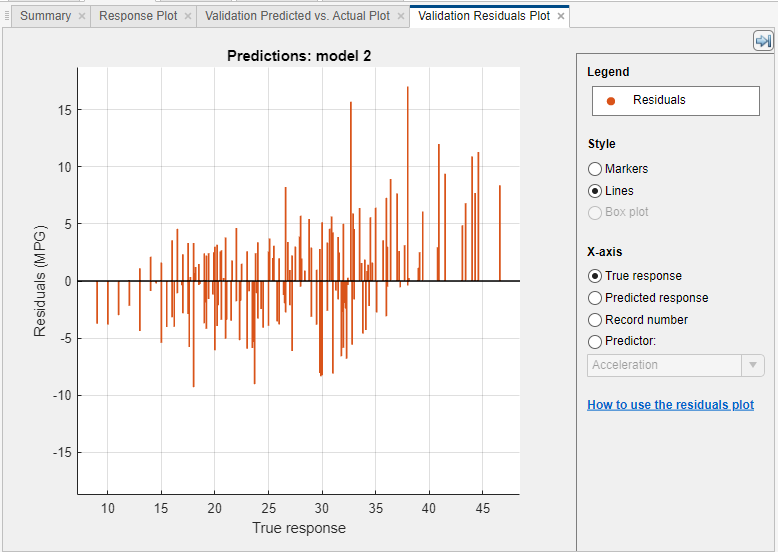Usually a good model has residuals scattered roughly symmetrically around 0. If you can see any clear patterns in the residuals, it is likely that you can improve your model.

17. To learn about model settings, choose the best model in the Models pane and view the advanced settings. The nonoptimizable model options in the Model Type gallery are preset starting points, and you can change additional settings. On the Regression Learner tab, in the Model Type section, click . Compare the different regression tree models in the Models pane, and observe the differences in the Advanced Regression Tree Options dialog box. The Minimum leaf size setting controls the size of the tree leaves, and through that the size and depth of the regression tree.

To try to improve the model further, change the Minimum leaf size setting to 8, and then train a new model by clicking .

View the settings for the selected trained model in the Current Model Summary pane or in the Advanced Regression Tree Options dialog box.

18. Export the selected model to the workspace. On the Regression Learner tab, in the Export section, click . In the Export Model dialog box, click to accept the default variable name `trainedModel`.

To see information about the results, look in the command window.

19. Use the exported model to make predictions on new data. For example, to make predictions for the `cartable` data in your workspace, enter:

`yfit = trainedModel.predictFcn(cartable)`
The output `yfit` contains the predicted response for each data point.

20. If you want to automate training the same model with new data or learn how to programmatically train regression models, you can generate code from the app. To generate code for the best trained model, on the Regression Learner tab, in the Export section, click .

The app generates code from your model and displays the file in the MATLAB Editor. To learn more, see Generate MATLAB Code to Train Model with New Data.

Tip

Use the same workflow as in this example to evaluate and compare the other regression model types you can train in Regression Learner.

Train all the nonoptimizable regression model presets available:

1. On the far right of the Model Type section, click the arrow to expand the list of regression models.

2. Click, and then click Train.To learn about other regression model types, see Train Regression Models in Regression Learner App.